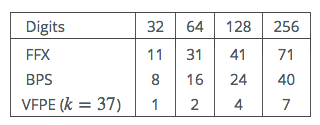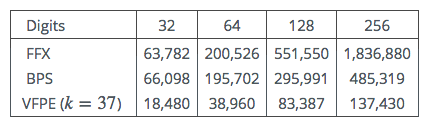# A Note on the Implementation of Format Preserving Encryption Modes

##### Dr Michael Scott

The American National Institute for Standards in Technology (NIST) is considering proposals for several modes of operation for Format Preserving Encryption (FPE). The idea behind FPE is quite simple: A plaintext should encipher to a ciphertext with exactly the same format and length. The classic example would be a credit card number, in which case the 18 decimal digit plaintext should encrypt to an 18 decimal digit ciphertext. This is clearly very convenient.

Here we implement three of the proposed modes, FFX proposed by Bellare, Rogaway and Spies, BPS proposed by Brier, Peyrin and Stern, and VFPE proposed by Sheets and Wagner. Details can be found on the NIST website.

We compare the modes for convenience of use, ease of implementation, and speed. The implementations described here will form a part of the MIRACL FPE toolkit.

### 1 Introduction

We do not labour the advantages of FPE. For certain applications it is clearly nice that the ciphertext has exactly the same form as the plaintext. Clearly the ciphertext can be stored and displayed in exactly the same format as the plaintext, and no special provision needs to be made for it. In legacy database applications a field for data which now needs to be encrypted, will not require any modification.

However getting FPE right is quite tricky, as the size of the plaintext can often be quite small. For each of the methods considered the authors have gone to some length to ensure the security of their solutions.

Each mode is based on a block cipher, and this can be chosen at will. Here we have used the Advanced Encryption Standard (AES) as the basis for all of our implementations. In the “real world” we imagine that most would do likewise.

The FFX mode from Bellare, Rogaway and Spies is probably the most complex, and clearly a lot of thought has gone into it. It has been through several iterations, the latest being FFX[Radix]. It is patented. Test vectors are supplied.

The BPS mode from Brier, Peyrin and Stern is unpatented. It comes in for some criticism from Bellare et al. for the way in which it deals with longer strings – in this case it is not a PRP – a pseudo-random permutation. However as they themselves point out long strings may not be appropriate for these FPE modes anyway, and BPS using the AES can for example handle strings of up to 56 decimal digits without invoking its longer strings mechanism, so in practice this may not be an issue. There are no test vectors provided.

The VFPE is a method invented by the Visa corporation, and is patented. It is the simplest mode considered here. There are no test vectors provided.

We assume that all of the proposals are equally secure (although this may not be the case – but such comparison is beyond the scope of this note).

### 2 General Implementation Issues

The absence of test vectors in two out of three cases is to be severely deprecated. Implementation from the provided descriptions is difficult enough even when one has test vectors to ensure correctness at each step. Without them it is impossible to be 100% sure that the implementation is correct. One common issue is how to present input for AES encryption. If a number is to be encrypted, should it be presented to the block cipher in big or little endian form? For example the AES accepts input as a block of 16 bytes, B, B etc. If the number 1 is to be encrypted should the AES input have B=1 or B=1? It would help considerably if authors were explicit about this.

### 3 Format Preserving Encryption

One of the challenges to be faced by the designer of an FPE mode is that the input strings can be quite small. Now the reason that a block cipher has a relatively large block size of typically 64 or 128 bits is to prevent a code book attack. Recall that a block cipher can be visualised as a massive fixed (and reversible) look-up table whereby the output ciphertext is a pseudo-random permutation of the input plaintext. There is a completely different look-up table for each possible key. Reductio ad absurdam – consider now a 1-bit block cipher with a 128-bit key. Clearly a 0 will consistently encipher as a 0 or a 1 for a fixed key. With one known bit of input and output the complete two-entry look-up table can be established and the system is broken – an attacker simply looks up each bit of ciphertext in the look-up table and ignores the key.

Therefore to work with a small block size a new idea is needed. One common idea is to make the block cipher “tweakable”. Each time a tweakable block cipher is tweaked, it in effect becomes a completely different block cipher. So if each encryption with the same key uses a different tweak, the result is a completely different ciphertext, and code book attacks are frustrated.

All of the modes considered here are tweakable, although VFPE achieves the same functionality by changing the counter mode initialisation vector (IV). As the strings to be encrypted get longer this tweakability becomes less important.

### 4 FFX mode

Although test vectors are provided, it was a struggle to interpret them. The first test vector appears to have been transcribed incorrectly. For example the provided P vector has only 15 entries – it should have 16. The input of length 10 is 0123456789. This converts to binary (in big endian form) as the two bytes 231,223. However test vector 2 has the same input, which this time converts to binary as 221,213. The latter appears correct to us, so we quickly gave up on test vector 1.

Test vector 2 worked smoothly. Test vector 3 included a tweak. However the tweak is handled as an ascii string of characters rather than as base 10 numbers as is the case for the input. Test Vector 4 is the only one to have an uneven input size. This is a case that needs to be handled carefully, as this situation can easily arise in practise. In fact the decryption algorithm as described in the paper is rather misleading. When the input is split A is the smaller part and B the bigger part – bigger by one. Then inside the loop A is assigned to B. This leaves the most significant element of B unassigned. Then A is updated. At this stage the most significant element of B should be updated from the most significant element of the new A.

Test vector 5 uses base 36, and the input is represented using the digits 0-9 followed by A-Z. These need to be carefully converted from ascii to base 36 numbers. With its larger input and tweak, this test requires an extra invocation of the AES algorithm when internally calculating the CBC-MAC.

Our implementation optimises as suggested by the authors by encrypting the CBC-MAC IV, the vector P, just once across all of the rounds. The authors appear concerned that “When the radix is not a power of two, the mod at line 38 may require significant attention”. We were puzzled by this remark, as we found this not to be an issue.

### 5 BPS mode

This mode is quite similar to FFX in that it also uses a Fesitel structure. Its advent seems to have motivated some of the recent changes to FFX. It was quite straightforward to implement, the only major issue being the lack of test vectors. There appears to be a small typo in the second last line of Algorithm 3, where$B{C}_{F,s,len,w}$ should be $B{C}_{F,s,ma{x}_{b},w}$. There is the same problem in algorithm 4.

The CBC mechanism for handling longer strings may raise doubts, but it is certainly convenient and requires less memory than FFX.

### 6 VFPE mode

This mode was probably the simplest to implement. It has a parameter$k$ which is basically the number of digits to the chosen base$n$ that are encrypted from each invocation of the AES. This should be a little less that the maximum possible, as for larger$k$ more ciphertext blocks might need to be rejected as they may introduce a bias. Blocks of$k$ random digits are generated using the AES in counter mode, which are then added modulo$n$ to each digit in the plaintext. The same random digits are subtracted to restore the plaintext on decryption. For base$n=10$ and using the AES,$k=37$ appears to be a good choice.

The only tricky part is determining the threshold for acceptability of AES ciphertexts. They must be less than ${n}^{k}.⌊{2}^{b}∕{n}^{k}⌋$. On the face of it, it might appear to require multi-precision arithmetic to calculate this threshold. The trick is to convert${2}^{b}$ to base$n$ by repeated division by $n$.

Then the threshold is simply this number with its last$k$ digits set to zero. Note that the AES ciphertext must be converted to base$n$ anyway for use in the encryption process, and comparison with the adjusted threshold is now trivial.

The counter used in the AES counter mode must be initialised externally. If the plaintext is greater than$k$ digits, as many invocations of the AES as are needed are carried out, incrementing the counter for each fresh block.

Note that VFPE is “malleable”, as it alone is based on a stream-cipher mode and does not use the Feistel structure of the other two modes. This makes it open to an active known plaintext substitution attack, and so some extra mechanism (typically a MAC) must be deployed to prevent this.

### 7 Timings

The timings will be roughly preportional to the number of AES encryptions required, and this in turn will be proportional to the number of digits in the plaintext and the number base. For FFX and BPS we assume a 64-bit tweak. We first count the number of AES encryptions in Table 1.

Table 1: Number of AES calls vs Number of decimal digitsAs can be seen FFX appears to be the most expensive, with VFPE the cheapest, with BPS in the middle

Actual timings in clock cycles were obtained using MIRACL’s internal AES code (which does not use Intel’s special AES instructions). The code was run on a single core of an Intel i5 520M, clocked at 2.4GHz. The time required for once-off AES key scheduling is not included.

Table 2: Clock cycles vs Number of decimal digitsSomewhat to our surprise the FFX code ties for speed with BPS for smaller input sizes. This is despite FFX using more Feistel rounds (10 as against 8).

### 8 Conclusions

Overall BPS mode would be our preference. It represents a compromise between the other two modes in terms of performance, and its unpatented status is a big attraction. Its method of dealing with longer strings may not be ideal, but in practise in the kind of applications where FPE is likely to be deployed, this will not be an issue. Recall that these modes can be used with any block cipher, and a block cipher with a larger block size is to be preferred with BPS. Here we note that the original Rijndael (which became the AES) can be used with a block size of 256 bits, which might be an option for BPS if it must be used with longer strings.